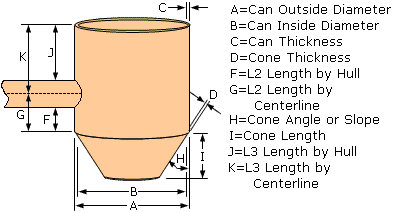# Can Rule Tab (Can Properties Dialog Box) - Intergraph Smart 3D - Help

## Intergraph Smart 3D Structure

PPMProduct
Intergraph Smart 3D
PPMCategory_custom
Help
Version_S3D
12.1(2019)

Specifies the properties for the selected can rule.

Diameter Rule

Select how you want to define the diameter of the tube. You can select:

• Outer Diameter - Select this option to match the outside diameter of the tube with the outside diameter of the supporting leg.

• Inner Diameter - Select this option to match the inside diameter of the tube with the inside diameter of the supporting leg.

• User Defined - Select this option to specify the diameter of the tube yourself. Cones are created if you define a diameter that is significantly larger than the cross-section of the supporting leg.

Can Outside Diameter

Specifies the outside diameter of the can.

Can Inside Diameter

Specifies the inside diameter of the can.

Can Thickness

Specifies the plate thickness of the can plate.

Cone 1 Thickness

Specifies the plate thickness of cone 1.

Cone 1 Material

Specifies the material for cone 1.

Specifies the material grade for cone 1.

Can Material

Specifies the material for the can.

Specifies the material grade for the can.

Minimum Extension Distance

Specify the minimum distance between the transverse member and the end of the can. If you type a distance that is less than the minimum extension distance that is defined in the Catalog, the software uses the distance defined in the Catalog, not the smaller distance that you specified here.

L2 Computation Method

Specifies how you want Smart 3D to measure the L2 minimum distance.

• If you select Hull-Factor, the software calculates the outside diameter of the can times the value you type in the L2 Factor box. The result is used as the minimum L2 distance, which is measured from the corresponding end of the can to the point on the transverse member hull closest to that end of the can.

• If you select Hull-Length, the software uses the distance you specify in the L2 Length box for L2, which is measured from the corresponding end of the can to the point on the transverse member hull closest to that end of the can.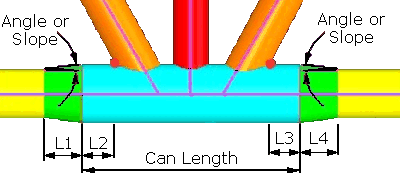L2 and L3 Lengths when using a Hull Extension Method

• If you select Centerline-Factor, the software calculates the outside diameter of the can times the value you type in the L2 Factor box. The result is used as the minimum L2 distance, which is measured from the corresponding end of the can to the centerline of the transverse member closest to that end of the can.

• If you select Centerline-Length, the software uses the distance you specify in the L2 Length box for L2, which is measured from the corresponding end of the can to the centerline of the transverse member closest to that end of can.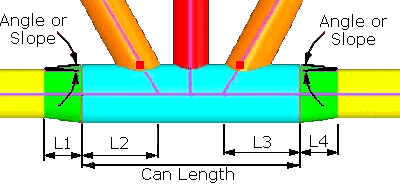L2 and L3 Lengths when using a Centerline Method

L2 Factor

Type the factor to use to calculate L2. The default is 0.25.

L2 Length

Type the L2 distance. This is the minimum distance that L2 can be. If there is no cone defined for that end of the can, then the L2 distance is the longer of the two chamfer lengths between the can and the support leg.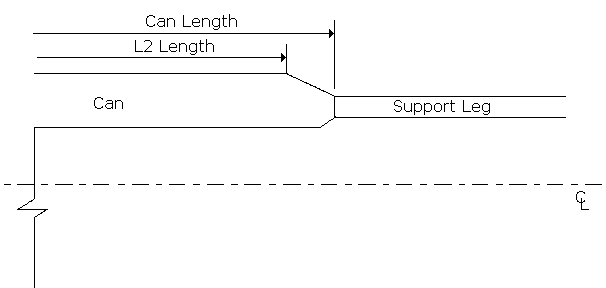L3 Computation Method

Specifies how you want Smart 3D to measure the L3 minimum distance.

• If you select Hull-Factor, the software calculates the outside diameter of the can times the value you type in the L3 Factor box. The result is used as the minimum L2 distance which is measured from the corresponding end of the can to the point on the transverse member hull closest to that end of the can.

• If you select Hull-Length, the software uses the distance you specify in the L3 Length box for L3, which is measured from the corresponding end of the can to the point on the transverse member hull closest to that end of the can.L2 and L3 Lengths when using a Hull Extension Method

• If you select Centerline-Factor, the software calculates the outside diameter of the can times the value you type in the L3 Factor box. The result is used as the minimum L3 distance, which is measured from the corresponding end of the can to the centerline of the transverse member closest to that end of the can.

• If you select Centerline-Length, the software uses the distance you specify in the L3 Length box for L3, which is measured from the corresponding end of the can to the centerline of the transverse member closest to that end of can.L2 and L3 Lengths when using a Centerline Method

L3 Length

Type the L3 distance. This is the minimum distance that L3 can be. If there is no cone defined for that end of the can, then the L3 distance is the longer of the two chamfer lengths between the can and the support leg (see L2 Length above for an illustration).

L3 Factor

Type the factor to use to calculate L3. The default is 0.25.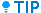Cone 1 is the cone nearest the start end of the supporting leg that the can is placed on.

Cone 1 Length Method

Specifies how you want to define the length of the first cone transition.

• If you select Angle, the length of the transition is determined by the geometry of the can diameter, the support member cross-section, and the angle specified in the Cone 1 Angle box. This is shown as Angle in the figures above.

• If you select Distance, the length of the transition is defined explicitly in the Cone 1 Length box. This distance is shown as L1 in the figures above.

• If you select Slope, the length of the transition is determined by the geometry of the can diameter, the support member cross-section size, and the slope specified in the Cone 1 Slope box. This is shown as Slope in the figures above.

Cone 1 Length

Specifies the length of the cone transition from the end of the support member to the start of the can tube. This distance is shown as L1 in the figures above. This option is available when Cone 1 Length Method is set to Distance.

Cone 1 Slope

Specifies the slope of the cone transition. The software does not create a cone if the slope you specify is greater than 100 (1/100) or less than 1-6. This option is available when Cone 1 Length Method is set to Slope.

Cone 1 Angle

Specifies the angle of the cone transition. The software does not create a cone if the angle you specify is less than 1 degree or greater than 89.0 degrees. This option is available when Cone 1 Length Method is set to Angle.Cone 2 is the cone nearest the end of the supporting leg that the can is placed on.

Cone 2 Length Method

Specifies how you want to define the length of the end cone transition.

• If you select Angle, the length of the transition is determined by the geometry of the can diameter, the support member cross-section, and the angle specified in the Cone 2 Angle box. This is shown as Angle in the figures above.

• If you select Distance, the length of the transition is defined explicitly in the Cone 2 Length box. This distance is shown as L4 in the figures above.

• If you select Slope, the length of the transition is determined by the geometry of the can diameter, the support member cross-section size, and the slope specified in the Cone 2 Slope box. This is shown as Slope in the figures above.

Cone 2 Length

Specifies the length of the cone transition from the end of the support member to the start of the can tube. This distance is shown as L4 in the figures above. This option is available when Cone 2 Length Method is set to Distance.

Cone 2 Slope

Specifies the slope of the cone transition. The software does not create a cone if the slope you specify is greater than 100 (1/100) or less than 1-6. This option is available when Cone 2 Length Method is set to Slope.

Cone 2 Angle

Specifies the angle of the cone transition. The software does not create a cone if the angle you specify is less than 1 degree or greater than 89.0 degrees. This option is available when Cone 2 Length Method is set to Angle.

Chamfer Slope

Specifies the slope of the chamfer located at the end of the can where the can's tube transitions into the can's cone. The chamfer length, which is determined by the defined sloped, contributes to the length of the can part of the overall can. The value you type here is used for both ends of the can.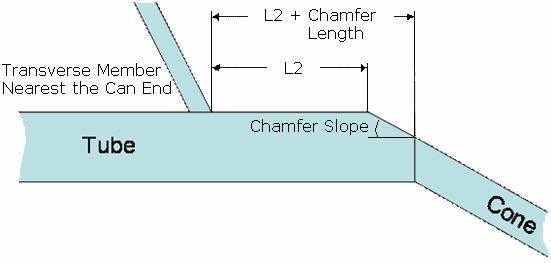Roundoff Distance

Type the distance to round off the total can length. The length of the can is rounded up to the next increment of this value.

Cone 2 Thickness

Specifies the plate thickness of cone 2.

Cone 2 Material

Specifies the plate material of cone 2.

Specifies the plate material grade of cone 2.

Inline Can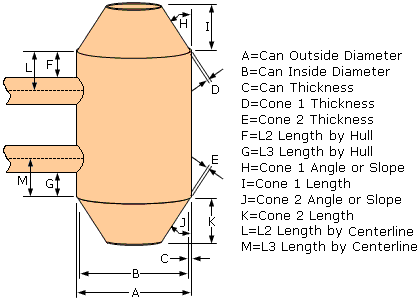Stub Can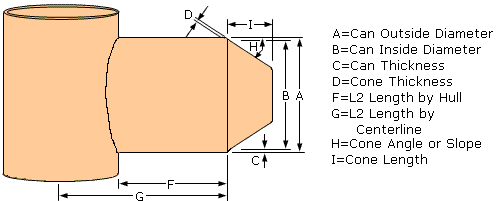End Can